Next: Green functions Up: Types of analysis Previous: Electromagnetism   Contents

### Sensitivity

A sensitivity analysis calculates how a variable G (called the objective function) changes with some other variables s (called the design variables), i.e. DG/Ds.

If s are the coordinates of some nodes, then the objective function usually takes the form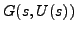, i.e. it is a direct function of the coordinates and it is a direct function of the displacements, which are again a function of the coordinates. One can write (vector- and matrix-denoting parentheses have been omitted; it is assumed that the reader knows that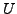and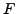are vectors,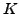and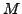are matrices and that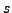and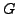are potentially vectors):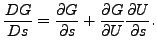(430)

The governing equation for static (linear and nonlinear) calculations is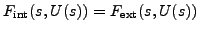, which leads to(431)

or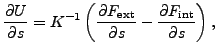(432)

where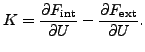(433)

Since for linear applications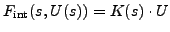and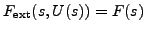, the above equations reduce in that case to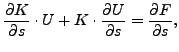(434)

or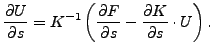(435)

Consequently one arives at the equation: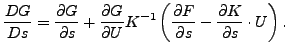(436)

For the speed-up of the calculations it is important to perform the calculation of the term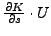on element level and to calculate the term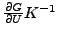before multiplying with the last term in brackets. Furhermore,should be calculated by solving an equation system and not by inverting.

For special objective functions this relationship is further simplified:

• ifis the mass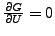.
• ifis the shape energy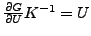.
• ifare the displacements Equation (435) applies directly

For eigenfrequencies as objective function one starts from the eigenvalue equation: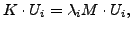(437)

from which one gets: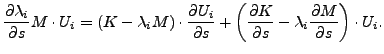(438)

Premultiplying with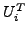and taking the eigenvalue equation and the normalization of the eigenvectors w.r.t.into account leads to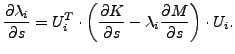(439)

Notice that this is the sensitivity of the eigenvalues, not of the eigenfrequencies (which are the square roots of the eigenvalues). This is exactly how it is implemented in CalculiX: you get in the output the sensitivity of the eigenvalues.

Subsequently, one can derive the eigenvalue equation to obtain the derivatives of the eigenvectors: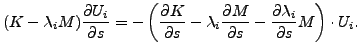(440)

If s is the orientation in some or all of the elements, the term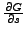is in addition zero in the above equations.

In CalculiX, G is defined with the keyword *OBJECTIVE, s is defined with the keyword DESIGNVARIABLES and a sensitivity analysis is introduced with the procedure card *SENSITIVITY.

If the parameter NLGEOM is not used on the *SENSITIVITY card, the calculation of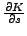does not contain the large deformation and stress stiffness, else it does. Similarly, without NLGEOMis calculated based on the linear strains, else the quadratic terms are taken into account.

If the objective function is the mass, the shapeenergy or the displacements a *STATIC step must have been performed. The displacementsand the stiffness matrixfrom this step are taken forandin Equation (436) (in the presence of a subsequent sensitivity stepis stored automatically in a file with the name jobname.stm). If the static step was calculated with NLGEOM, so should the sensitivity step in order to be consistent. So the procedure cards should run like:

*STEP
*STATIC
...
*STEP
*SENSITIVITY
...


or

*STEP,NLGEOM
*STATIC
...
*STEP,NLGEOM
*SENSITIVITY
...


If the objective functions are the eigenfrequencies (which include the eigenmodes), a *FREQUENCY step must have been performed with STORAGE=YES. This frequency step may be a perturbation step, in which case it is preceded by a static step. The displacements, the stiffness matrixand the mass matrixfor equations (439) and (440) are taken from the frequency step. If the frequency step is performed as a perturbation step, the sensitivity step should be performed with NLGEOM, else it is not necessary. So the procedure cards should run like:

*STEP
*FREQUENCY,STORAGE=YES
...
*STEP
*SENSITIVITY
...


or

*STEP
*STATIC
...
*STEP,PERTURBATION
*FREQUENCY,STORAGE=YES
...
*STEP,NLGEOM
*SENSITIVITY
...


or

*STEP,NLGEOM
*STATIC
...
*STEP,PERTURBATION
*FREQUENCY,STORAGE=YES
...
*STEP,NLGEOM
*SENSITIVITY
...


(a perturbation frequency step only makes sense with a preceding static step).

The output of a sensitivity calculation is stored as follows (frd-output only if the SEN output request was specified underneath a *NODE FILE card):

For TYPE=COORDINATE design variables the results of the target functions MASS, SHAPE ENERGY, EIGENFREQUENCY and DISPLACEMENT (i.e. the sum of the squares of the displacements in all objective nodes) are stored in the .frd-file and can be visualized using CalculiX GraphiX.

For TYPE=ORIENTATION design variables the eigenfrequency sensitivity is stored in the .dat file whereas the displacement sensitivity (i.e. the derivative of the displacements in all nodes w.r.t. the orientation) is stored in the .frd-file. The order of the design variables is listed in the .dat-file. All orientations defined by *ORIENTATION cards are varied, each orientation is defined by 3 independent variables. So for n *ORIENTATION cards there are 3n design variables. The sensitivity of the mass w.r.t. the orientation is zero.

Finally, it is important to know that a sensitivity analysis in CalculiX only works for true 3D-elements (no shells, beams, plane stress, etc...).Next: Green functions Up: Types of analysis Previous: Electromagnetism   Contents
guido dhondt 2018-12-15